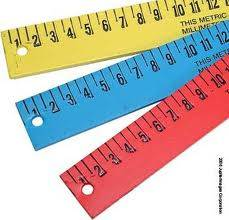# 1 Cents Equals How Many Square Meters?

Cent is a land measurement from older times in India.

An acre = 4840 square yards which in turn = 43560 square feet

As everyone knows, in monetary terms there are 100 cents to one dollar. As with that 100 cents = one acre.

One cent = 1/100th of an acre

So one cent = 40.46856 which is multiplied with the unit (or units) of reference (in this case meters squared) to produce the units to measure the area.Of course these days most countries measure areas of land in acres. One acre is equivalent to 43,560 square feet. It may be easier to visualise an acre if you think of it as 80% of the size of the entire size of an American football field (including the end zones)

The word 'acre' comes from the the old English word "aecer" meaning "open field".

thanked the writer.